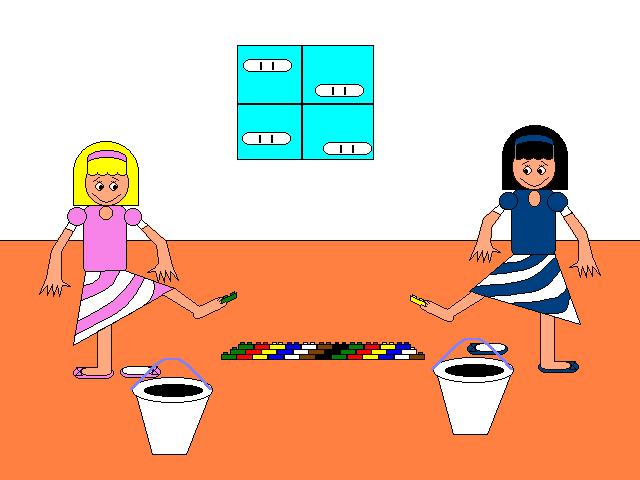#### Difficulty: MediumWhen Candy & Fudge were 9-year-olds, they decided to build a lego pyramid. Here are some interesting questions to answer:

1. What's the probability that Candy or Fudge will take a black lego from their buckets?
2. What will be the color of the lego on top?
3. How many legoes have they connected so far?
4. How many will they have to use?
5. How many rows will their pyramid have?

Hints:

1. How many colors are seen on the legoes?
2. If the color of the legoes closest to Candy are ______, then the last one will be ______.
3. Count. Just Count. (I suggest you count by multiples of 3!)
4. 1+2+3+...+(the number of legoes on the bottom row)=(the total number of legoes.)
5. (The number of legoes on the bottom row)=(the total number of rows the pyramid will have.)